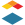# [New Quizzes] Multiple Choice Questions using Formulas/Variables

Problem statement:

In Canvas it is not possible to include dynamic variables in multiple choice questions (or any other question type besides Formula). The use of dynamic variables would allow instructors to write one question that could be presented with different values to every student.

Proposed solution:

Allow the use of formulas in multiple choice questions, both in the stem and the responses. For example, a question could be written like: "If 2/3 lb of tomatoes costs [price], how much does one pound of tomatoes cost?" and the choices would be a. [price]*2 b. [price]*(2/3) c. [price]* (3/2) d. [price]*3 This would save time creating multiple similar versions of a question.

User role(s):